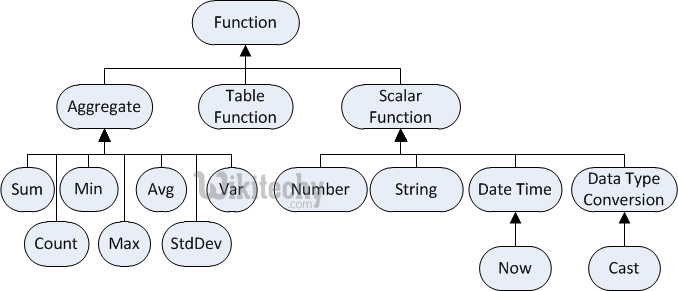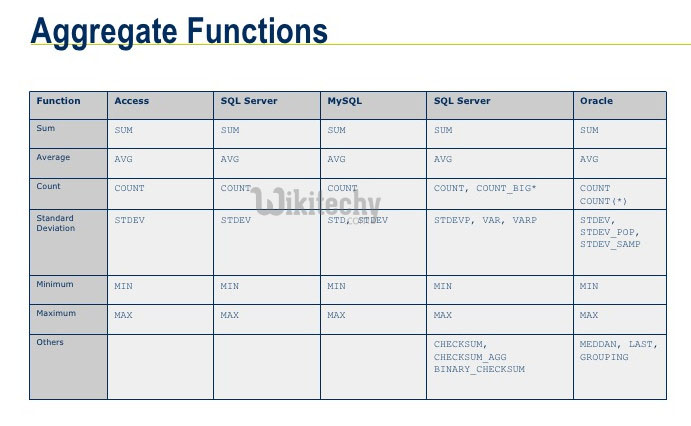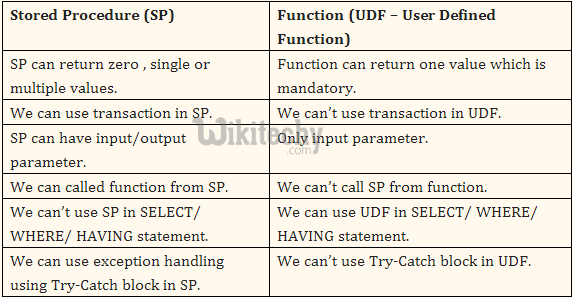# Functions in sql | Functions command in sql - sql - sql tutorial - learn sql• A user-defined function is a Transact-SQL or common language runtime (CLR) routine that accepts parameters, performs an action, such as a complex calculation, and returns the result of that action as a value.
• The return value can either be a scalar (single) value or a table.
• Since we have started dealing with numbers, the next question to ask is if it is possible to do math on those numbers, such as summing them up or taking their average.

## What is the use of function in SQL?

• A user-defined function is a Transact-SQL or common language runtime (CLR) routine that accepts parameters, performs an action, such as a complex calculation, and returns the result of that action as a value. The return value can either be a scalar (single) value or a table.

## SQL has several arithmetic functions, and they are:

SQL Function Description
AVG Average of the column.
COUNT Number of records.
MAX Maximum of the column.
MIN Minimum of the column.
SUM Sum of the column.
ROUND Round a number to s specified precision.
• The syntax for using functions is,

## Aggregate Functions in SQL## SQL COUNT ():

• This function returns the number of rows in the table that satisfies the condition specified in the WHERE condition.
• If the WHERE condition is not specified, then the query returns the total number of rows in the table.

## Example:

• If you want the number of employees in a particular department, the query would be:
• The output would be '2' rows.
• If you want the total number of employees in all the department, the query would take the form:
• The output would be '5' rows.

## SQL DISTINCT():

• This function is used to select the distinct rows.

## Example:

• If you want to select all distinct department names from employee table, the query would be:
• To get the count of employees with unique name, the query would be:

## SQL MAX():

• This function is used to get the maximum value from a column.
• To get the maximum salary drawn by an employee, the query would be:

## SQL MIN():

• This function is used to get the minimum value from a column.
• To get the minimum salary drawn by an employee, he query would be:

## SQL AVG():

• This function is used to get the average value of a numeric column.
• To get the average salary, the query would be

## SQL SUM():

• This function is used to get the sum of a numeric column
• To get the total salary given out to the employees,

## Stored Procedure VS Functions